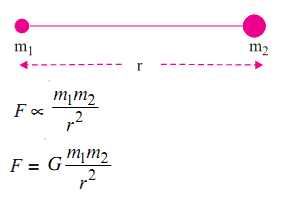Gravitation

While Newton was sitting under an apple tree, an apple fell on him. The fall of the apple set Newton thinking, why did the apple fall down? If some force is acting on the apple then it must be in accelerated motion.

Newton knew that bodies fall towards the earth due to force of gravity. He further thought, if the earth can attract an apple or a stone, can it also attract the moon? He was also curious to know whether the same force was responsible for keeping the planets go around the sun in their orbits.

Newton concluded that in order to move in a circular orbit the moon must be attracted by the earth continuously. Arguing in the same lines he said that there exists a force between the sun and the planets. The force is known as the gravitational force. He stated that gravitational force exists everywhere in the universe. All objects in the universe attract each other.

Newton's Law of Gravitation

Every particle in the universe attracts every other particle with a force. This force is proportional to the product of their masses and inversely proportional to the square of the distance between them. The force is along the line joining the two particles.G is a constant of proportionality. It is called the universal gravitational constant. Its value is same everywhere on the earth or in the universe.

G = 6.67 × 10-11 Nm2kg-2

As the value of G is very small, the force of gravitation between objects of ordinary mass is very weak.

Acceleration due to Gravity

The acceleration due to earth’s gravity is denoted by g.

F = mg = GMm/r2

g = GM/r2

M is the mass of the earth and r is the distance between the object and the centre of the earth. If the object is on or near the surface of the earth, the distance r in equation will be equal to the radius of the earth R.

g = GM/R2

The value of g is independent of the mass of the freely falling body.

g = 9.8 ms-2

Variation in g

On the surface of earth the value of g is minimum at the equator. It increases on moving towards the poles and is maximum at the poles.

The value of g decreases on moving above or below the surface of earth. It is zero at the centre of the earth.

Mass and Weight

Mass

Mass of a body is the quantity of matter contained in the body. Mass of an object is constant and does not change from place to place. It remains the same whether the object is on earth, on moon or anywhere in outer space. The mass of an object is measured with the help of a pan balance.

Weight

The weight of an object is the force with which it is attracted towards the earth.

Force = Mass × Acceleration

F = mg

If weight of an object is denoted by W, then

W = mg

This force (weight) acts vertically downwards. It has both magnitude and direction. The weight of an object is generally measured by a spring balance.

Kepler's Laws

(i) All planets move around the sun in elliptical orbits having the sun at one focus of the orbit.

(ii) The areal speed of the planet remains constant.

(iii) The square of the period of revolution of any planet around the sun is directly proportional to the cube of its mean distance from the sun.

T2 ∝ r3

Satellite

A heavenly body revolving around a planet in an orbit is called natural satellite. For example, moon is the natural satellite of the earth.

Orbital Velocity of Satellite

The speed of satellite depends upon its height from the earth's surface. The farther is the satellite from the earth's surface, the lesser will be its speed.

The velocity of a satellite does not depend upon its mass. Therefore, if various satellites of different masses revolve around the earth in same orbit then their orbital velocities
will be the same.

The orbital velocity of a satellite which orbits very close to the earth's surface is 8 km/sec.

Period of Revolution of Satellite

The period of revolution of a satellite depends upon its height from the earth's surface. The farther is the satellite from the earth's surface the higher will be its period of revolution.

The period of revolution of a satellite does not depend upon its mass. The periods of revolution of different satellites of different masses revolving around the earth in same orbit will be equal.

The period of revolution of a satellite orbiting very close to earth is 5063 second or 84 min (approx).

Communication Satellite

If the period of revolution of an artificial satellite revolving around the earth in the equatorial plane (normal to the axis of rotation of the earth) from west to east is equal to the period of rotation of earth about its own axis (24 hours), the satellite will always appear stationary from any place in the equatorial plane.

Such a satellite is used for communication purposes. It is also called geostationary or synchronous satellite.

Escape Velocity

The escape velocity from the earth's surface is 11.2 km/sec.

Relation Between Orbital Velocity and Escape Velocity

If the orbital velocity of an earth's satellite increases to √2 times (increases by 41.4%) then the satellite will leave its orbit and escape into space.